# To estimate: The value of f ′ ( − 3 ) using the graph of f.### Single Variable Calculus: Concepts...

4th Edition
James Stewart
Publisher: Cengage Learning
ISBN: 9781337687805### Single Variable Calculus: Concepts...

4th Edition
James Stewart
Publisher: Cengage Learning
ISBN: 9781337687805

#### Solutions

Chapter 2.7, Problem 1E

(a)

To determine

## To estimate: The value of f′(−3) using the graph of f.

Expert Solution

The value of f(3) is 0.2.

### Explanation of Solution

Estimation:

Draw the slope of the tangent at the point x=3.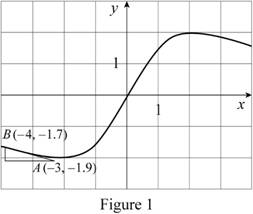The calculation of the slope at x=3 is as follows,

From the Figure 1,

m=1.9+1.73+4=0.2

Thus, f(3)=0.2.

(b)

To determine

### To estimate: The value of f′(−2) using the graph of f.

Expert Solution

The value of f(2) is 0.

### Explanation of Solution

Estimation:

Draw the slope of the tangent at the point x=2.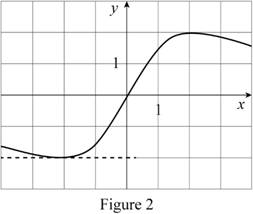From the Figure 2, tt is clear that the tangent to the graph at x=2 is horizontal.

Thus, f(2)=0

(c)

To determine

### To estimate: The value of f′(−1) using the graph of f.

Expert Solution

The value of f(1) is 1.

### Explanation of Solution

Estimation:

Draw the slope of the tangent at the point x=1.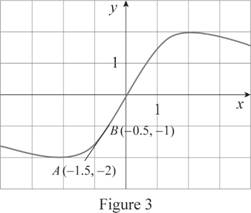From the Figure 3, Slope of AB

m=1+20.5+1.5=1

Thus, f(1)=1.

(d)

To determine

### To estimate: The value of f′(0) using the graph of f.

Expert Solution

The value of f(0) is 2.

### Explanation of Solution

Estimation:

Draw the slope of the tangent at the point x=0.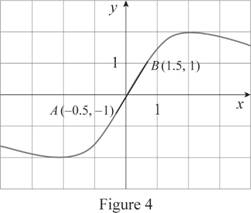From the Figure 4, Slope of AB

m=110.51.5=2

Thus, f(0)=2.

(e)

To determine

### To estimate: The value of f′(1) using the graph of f.

Expert Solution

The value of f(1) is 1.

### Explanation of Solution

Estimation:

Draw the slope of the tangent at the point x=1.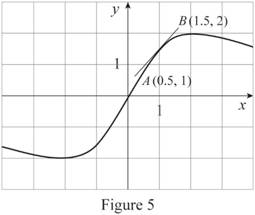From the Figure 5, Slope of AB

m=211.50.5=1

Thus, f(1)=1.

(f)

To determine

### To estimate: The value of f′(2) using the graph of f.

Expert Solution

The value of f(2) is 0.

### Explanation of Solution

Estimation:

Draw the slope of the tangent at the point x=2.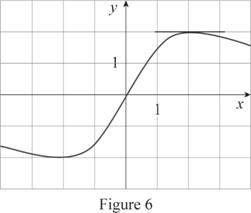From the Figure 6, it is clear that the tangent to the graph at x=2 is horizontal.

Thus, f(2)=0.

(g)

To determine

### To estimate: The value of f′(3) using the graph of f.

Expert Solution

The value of f(3) is 0.2.

### Explanation of Solution

Estimation:

Draw the slope of the tangent at the point x=3.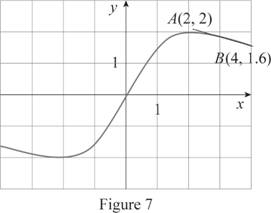From the Figure 7, Slope of AB

m=1.6242=0.2

Thus, f(3)=0.2.

To Sketch the graph of f, use the information from above parts to draw the graph of f(x) as shown in Figure 8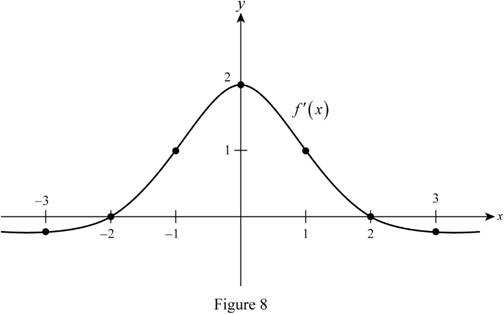From Figure 1, it is observed that the graph of f(x) is an even function.

### Have a homework question?

Subscribe to bartleby learn! Ask subject matter experts 30 homework questions each month. Plus, you’ll have access to millions of step-by-step textbook answers!